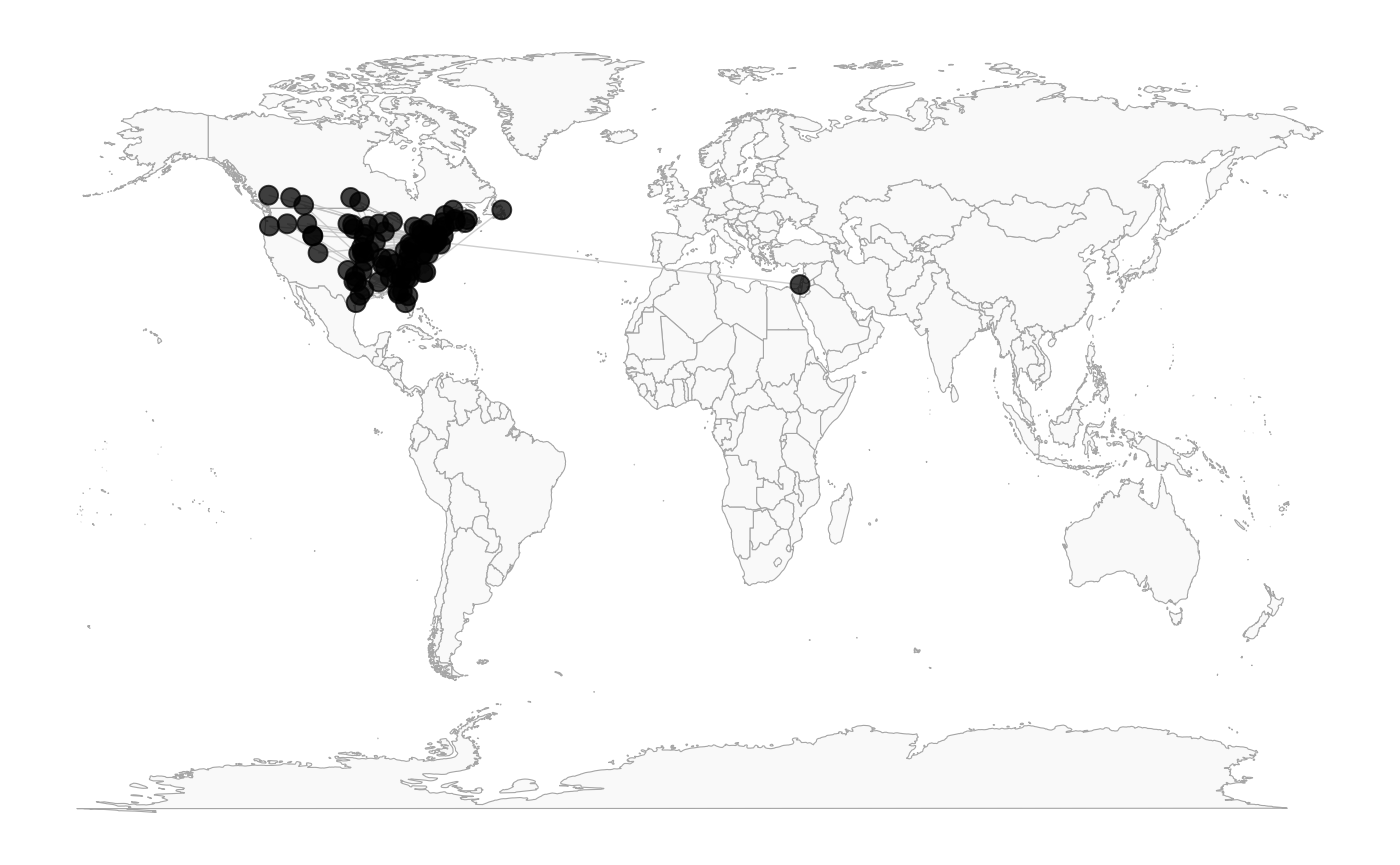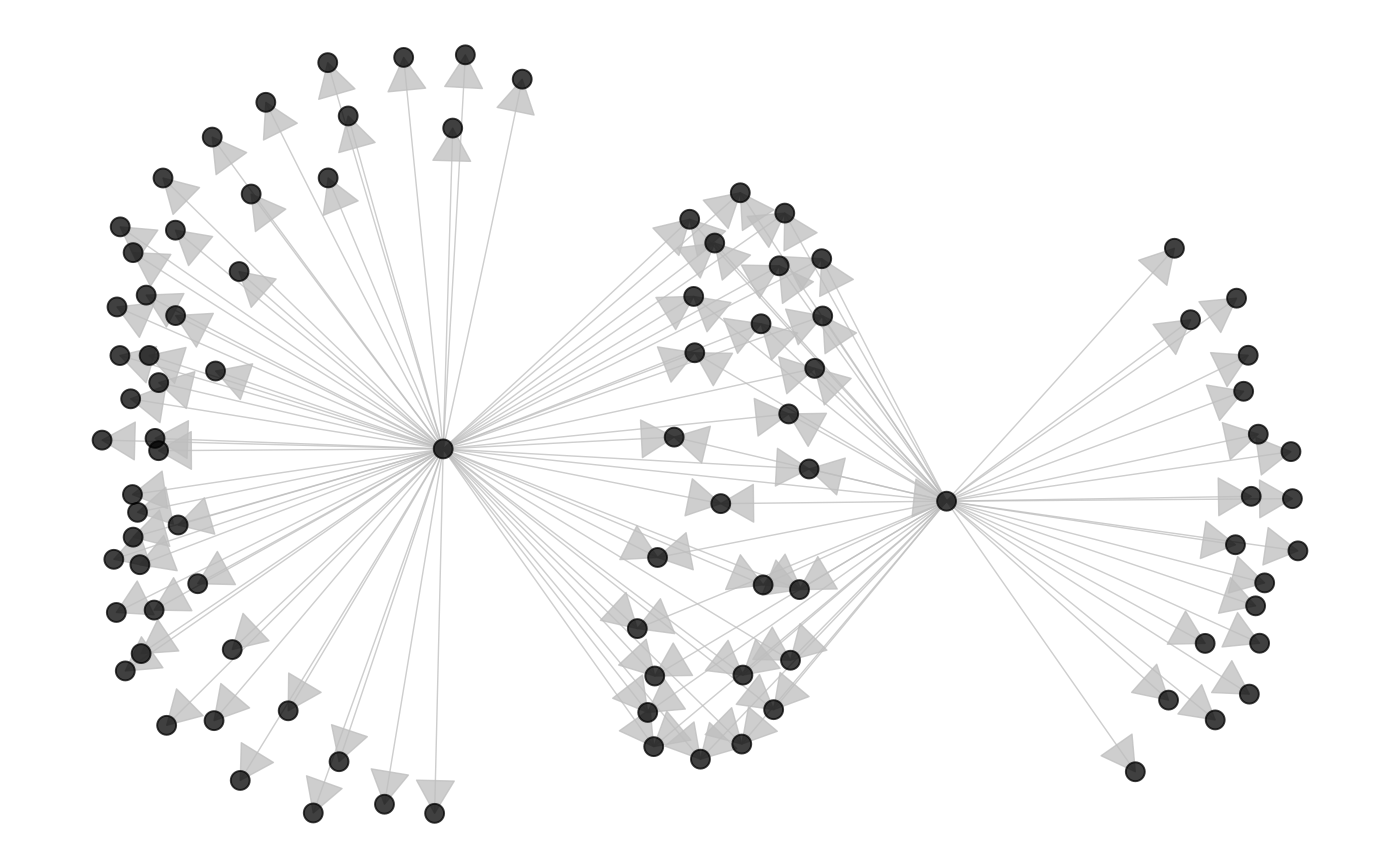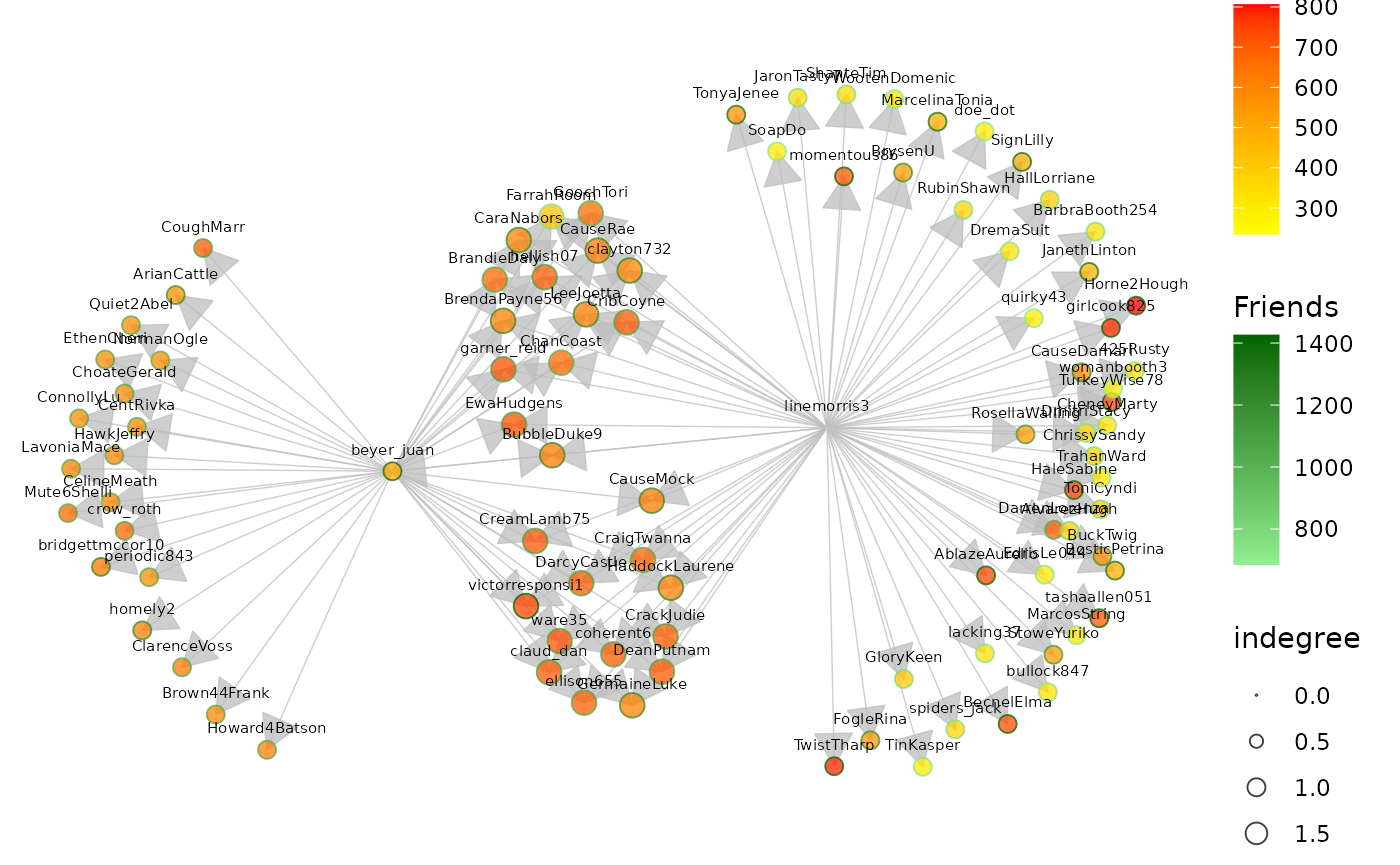library(GGally)
#> Registered S3 method overwritten by 'GGally':
#>   method from
#>   +.gg   ggplot2

# GGally::ggnetworkmap()

ggnetworkmap() is a function for plotting elegant maps using ggplot2. It builds on ggnet() by allowing to draw a network over a map, and is particularly intended for use with ggmap.

## Example: US airports

This example is based on a tutorial by Nathan Yau at Flowing Data.

suppressMessages(library(ggplot2))
suppressMessages(library(maps))
suppressMessages(library(network))
suppressMessages(library(sna))

rownames(airports) <- airports$iata # select some random flights set.seed(123) flights <- data.frame( origin = sample(airports[200:400, ]$iata, 200, replace = TRUE),
destination = sample(airports[200:400, ]$iata, 200, replace = TRUE) ) # convert to network flights <- network(flights, directed = TRUE) # add geographic coordinates flights %v% "lat" <- airports[ network.vertex.names(flights), "lat" ] flights %v% "lon" <- airports[ network.vertex.names(flights), "long" ] # drop isolated airports delete.vertices(flights, which(degree(flights) < 2)) # compute degree centrality flights %v% "degree" <- degree(flights, gmode = "digraph") # add random groups flights %v% "mygroup" <- sample(letters[1:4], network.size(flights), replace = TRUE) # create a map of the USA usa <- ggplot(map_data("usa"), aes(x = long, y = lat)) + geom_polygon(aes(group = group), color = "grey65", fill = "#f9f9f9", size = 0.2) # trim flights delete.vertices(flights, which(flights %v% "lon" < min(usa$data$long))) delete.vertices(flights, which(flights %v% "lon" > max(usa$data$long))) delete.vertices(flights, which(flights %v% "lat" < min(usa$data$lat))) delete.vertices(flights, which(flights %v% "lat" > max(usa$data\$lat)))

# overlay network data to map
ggnetworkmap(usa, flights, size = 4, great.circles = TRUE,
node.group = mygroup, segment.color = "steelblue",
ring.group = degree, weight = degree)This next example uses data from a Twitter spam community identified while exploring and trying to clear-up a group of tweets. After coloring the nodes based on their centrality, the odd structure stood out clearly.

data(twitter_spambots)
# create a world map
world <- fortify(map("world", plot = FALSE, fill = TRUE))
world <- ggplot(world, aes(x = long, y = lat)) +
geom_polygon(aes(group = group), color = "grey65",
fill = "#f9f9f9", size = 0.2)

# view global structure
ggnetworkmap(world, twitter_spambots)Is the network really concentrated in the U.S.? Probably not. One of the odd things about the network, is a much higher proportion of the users gave locations that could be geocoded, than Twitter users generally.

Let’s see the network topology

ggnetworkmap(net = twitter_spambots, arrow.size = 0.5)Coloring nodes according to degree centrality can highlight network structures.

# compute indegree and outdegree centrality

arrow.size = 0.5,
node.group = indegree,
ring.group = outdegree, size = 4) +
scale_fill_continuous("Indegree", high = "red", low = "yellow") +
labs(color = "Outdegree")Some Twitter attributes have been included as vertex attributes.

# show some vertex attributes associated with each account
scale_color_continuous(low = "lightgreen", high = "darkgreen")